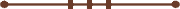####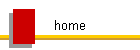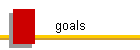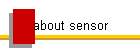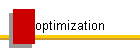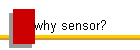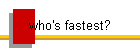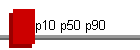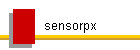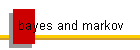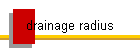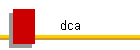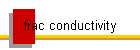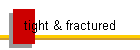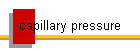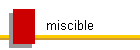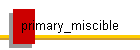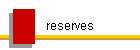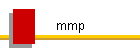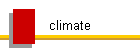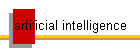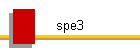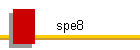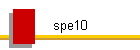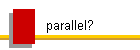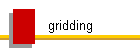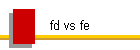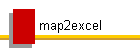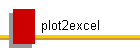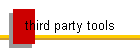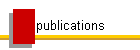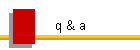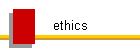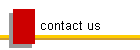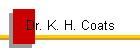#### Uncertainty Quantification - P10 / P50 / P90*

Also see:

When there are significant uncertainties in our heterogeneous reservoir descriptions, as there almost always are, there is no such thing as a meaningful P10, P50, P90, or Px case description.  There are only meaningful P10, P50, or Px results that must be determined from probabilistic analysis.  Any valid question in reservoir modeling, regardless of the model used, must be asked to some number of cases, representing many combinations of the uncertainties, in order to obtain a probabilistic distribution of the answer.   Absolute predictions require a statistically significant set of cases, but optimizations may require only a small number (see SensorPx Example 3). Individual scenarios have a near-zero probability of occurrence, and any desired number of Px cases of oil recovery, for example, can be found or constructed.  When the uncertainties have large effects on the results, no single case can answer any question or represent any probability of description or behavior, and is virtually meaningless in itself, beyond the Px probabilistic result that it reproduces and that was used to choose it amongst the considered scenarios.

In probabilistic analysis, one might run 1000 realizations of equally-probable combinations of the uncertain variables.  The estimated P90 oil recovery is the recovery that is exceeded in 90% of the cases (there is a 90% chance the recovery will be greater than the exceedance P90 value)**.  "Cumulative" probabilities are the opposite of exceedance probabilities:

low            median           high

Exceedance probabilities:          P90               P50             P10

Cumulative probabilities:            P10               P50             P90

A given case might give a P90 oil recovery and a P10 gas recovery.  In general there will be no realization that gives P90 results for more than one variable.  Depending on how much effect the uncertain variables have on the results, there may be no such thing as "what happens in the neighborhood of the P10".  There is such a thing, if the effect is little.  Two P90 cases (of a given variable) can easily be absolutely and completely different.  Multiple P10 cases tend to exhibit less difference in description and behavior.  The differences in multiple cases giving a result of the same exceedance probability Px decrease with decreasing value of x.

Consider a case that gives (optimistic) P10 oil recovery that has very many wells.  From that case one might easily construct two P90 oil recovery (pessimistic) cases that each operate a completely different subset of the wells in the P10 oil recovery case.

That same extreme difference of representative cases naturally results from stochastic (or probabilistic) representation of the most common uncertain variables, porosity and permeability.

Coming up with a Px case requires a reverse model calculation, no matter what model is used, to determine the inputs corresponding to a set of Px scalar outputs, as a function of time.  That is a far, far more complex task than the forward model.  Those companies and processes insisting on single or a few representative probabilistic cases (rather than results) are not correctly using reservoir models.

Providing a number is never a problem, as an answer to a valid question.  The answer to any question is always “the most probable answer is …”, or “the mean and standard deviation are …” or “this is the estimated probabilistic distribution of the prediction or benefit”, or "the answer can not be determined by reservoir modeling".  Only  probabilistic outcomes, or results, can be reliably determined for partially unknown systems, in reserves or prospect evaluation, and even in history matching and optimization.  The huge problem arises in reservoir modeling when representative probabilistic cases are required or perceived to be needed or meaningful, because in general no such meaningful cases exist, and in general it is extremely unlikely to identify any meaningful individual “realisations that are near the P90/50/10" as some suggest. In the typical case we might run thousands of realizations in an uncertainty space characterized by infinite numbers of real possibilities, but only billions or trillions or very many more restricted possibilities (the number of restricted possibilities is M**n where M is the number of discrete values allowed and n is the number of variables) .  For example, if there are 1000 variables and 10 allowed values for each, then the number of restricted possibilities is 10**1000.  If 1000 realizations are run, and then another 1000, then the probability is near zero that any found Px case in the first 1000 runs will be similar to any found in the second set, perhaps for all x but at least for x >> 0.

The same principles apply using any model with uncertain inputs that is used to obtain a probabilistic solution, which includes the use of analogs and experience.  For example, unless you have production, initial well rate and decline curve parameters are always uncertain.  Some number of analogs and amounts of experience and data allow engineers to compare wells with ranked analog results and estimate probabilistic results from them.  The uncertainty decreases with increasing applicable analogs, experience, and data.  The applicable analogs are simply the realizations of the uncertainties.  Local similarities in field geology and production behavior are learned from experience and significantly reduce uncertainty.

Simulation can further reduce uncertainty by relating production and injection to input descriptive properties of the reservoir(s), well(s), facilities, and fluids, and initial conditions and boundary conditions versus time.  But much of that input data is potentially uncertain, especially in optimizations, when we are attempting to determine optimal values of the input controls like well locations and completions and constraints (process).  Simulation can be used to numerically quantify the uncertainty in predicted production and injection, and to probabilistically optimize it, as a function of uncertainty in input descriptive variables and options in control variables.  Simulation is needed when it becomes impossible to otherwise predict the effects of heterogeneous reservoir/well descriptive variables and/or controls on production and recovery.

Spe11 makes a good example.  It is a well-known 10x10x3 blackoil model of gas injection and oil recovery, with the single gas injector completed in (1,1,1) and the single producer in (10,10,3). Layer thicknesses are 20, 30, and 50 ft, respectively.  Change the bhp of the gas injection well from 10000 to 9000 psi (to avoid effects of the negative compressibility error in the specified pvt data).  Also complete both the injector and producer in all 3 layers, and change Kz to be equal to .1*Kx.  The Sensor datafile is spe1pbase.dat.

Assume that the base case is our "best guess" case, which is defined by the most likely values of all uncertain inputs, and that only the areal layer permeabilities K1 K2 K3 are uncertain.  Base case values are 500, 50, and 200 md respectively.  Assume that the base case layer perms are estimated from analogs are not in error by more than a factor of 2 in either direction, i.e. 250 < K1 < 1000, 25 < K2 < 100, and 100 < K3 < 400, and that the probability distribution is uniform (usually permeability will follow a log-normal distribution).

Results, verifiable with any simulator (they should be the same or very close, for the given individual cases):

The base case gives cumulative oil recovery of 20.02%.

The Sensor data file spe1p.dat specifies the above permeability ranges as uniform random distributions.  Each execution of spe1p.dat gives a different and equally probable realization of the 3 unknowns.  The Makespx datafile spe1p.mspx specifies that SensorPx will compute P10, P50, and P90 results from the output of 10,000 runs of spe1p.dat that will be made in 8 simultaneous processes of 1250 serial runs each  It was found that sets of 1000, 3000, and 5000 runs were not sufficient to provide accurate and reproducible probabilistic results, but 10,000 runs yields P10, P50, and P90 phase rates and cumulatives that are reproducible within 1%, for the given uncertainties, in about 6 minutes on our machine.  The large number of runs required to obtain a statistically significant set, for only 3 total variables, is due to the fact that the variables have very large effects on results over their given ranges.  In another example using different assumptions of uncertainty (link to SensorPx Example 1 is at bottom of this page), 10,000 runs was found to be sufficient to quantify uncertainty in results for 900 uncertain variables that are randomly populated according to their input probability distributions.

The calculated Field Px,y,z values are given in the SensorPx output file spe1p.log.  Results at end of run are:

FINAL FIELD RESULTS

VARIABLE      TIME              MIN             PZ              PY              PX              MAX

(CASE)          (CASE)          (CASE)          (CASE)          (CASE)

--------------------------------------------------------------------------------------------------------

QOIL       0.3650000E+04   0.1648364E+04   0.3586624E+04   0.8808262E+04   0.2000000E+05   0.2106339E+05

2669            5593            5373            4780            2529

QWAT       0.3650000E+04   0.0000000E+00   0.0000000E+00   0.0000000E+00   0.0000000E+00   0.0000000E+00

0               0               0               0               0

QGAS       0.3650000E+04   0.3814416E+05   0.1226484E+06   0.1516267E+06   0.1977881E+06   0.2954274E+06

3091            8075            2262            7568            1276

QWI        0.3650000E+04   0.0000000E+00   0.0000000E+00   0.0000000E+00   0.0000000E+00   0.0000000E+00

0               0               0               0               0

QGI        0.3650000E+04   0.8418205E+05   0.1000000E+06   0.1000000E+06   0.1000000E+06   0.1000460E+06

3091            3125            5333            4631            2886

WCUT       0.3650000E+04   0.0000000E+00   0.0000000E+00   0.0000000E+00   0.0000000E+00   0.0000000E+00

0               0               0               0               0

GOR        0.3650000E+04   0.1907208E+04   0.6884886E+04   0.1898299E+05   0.3733397E+05   0.7145449E+05

3091            4980            4635            3583            2669

CUMOIL     0.3650000E+04   0.3931248E+05   0.4819892E+05   0.6493193E+05   0.7300000E+05   0.7305285E+05

5833            5860            3101            1888            3051

CUMWAT     0.3650000E+04   0.0000000E+00   0.0000000E+00   0.0000000E+00   0.0000000E+00   0.0000000E+00

0               0               0               0               0

CUMGAS     0.3650000E+04   0.9493449E+05   0.2111026E+06   0.4339683E+06   0.5052956E+06   0.5260374E+06

3091            4082            9117            3805             949

CUMWI      0.3650000E+04   0.0000000E+00   0.0000000E+00   0.0000000E+00   0.0000000E+00   0.0000000E+00

0               0               0               0               0

CUMGI      0.3650000E+04   0.3511303E+06   0.3650000E+06   0.3650000E+06   0.3650000E+06   0.3650000E+06

3734            2399            5181            8195            2486

OILREC     0.3650000E+04   0.1382300E+02   0.1694764E+02   0.2283128E+02   0.2566817E+02   0.2568675E+02

5833            5860            3101            1888            3051

GASREC     0.3650000E+04  -0.7122196E+02  -0.4260883E+02   0.1909491E+02   0.3884295E+02   0.4458563E+02

5832            4082            9117            3805             949

PAVGHC     0.3650000E+04   0.1693110E+04   0.1883883E+04   0.2642994E+04   0.6125840E+04   0.8657533E+04

949            2484            6907            6222            3091

PAVG       0.3650000E+04   0.1693103E+04   0.1883872E+04   0.2642970E+04   0.6125770E+04   0.8657509E+04

949            2484            6907            6222            3091

The P50 oil recovery (PY OILREC) is given as 22.83%.  Note that each Px, Py, and Pz result in the entire table is from a different case (the case number giving each result is indicated below the result).  Exceptions are pairs of variables that can be essentially the same or are strongly related, like PAVG and PAVGHC, and OILREC and CUMOIL. For example, of all runs, case number 3091 in the above table has the lowest cumulative gas production and final producing GOR, and the highest final average pressure (all related to very low gas production).  But in general there is no such thing as a Px, Py, or Pz case.  The case that was found to give that 22.83% P50 oil recovery is shown in the results table to be case number 3101 (case3101.out), which has K1,K2,K3 values of 908.13, 45.01, and 343.31 md, respectively.

We can easily modify the base case (K1,K2,K3 = 500,50,200, oil recovery = 20.02%) by manually changing layer permeabilities in very different ways that will give that same P50 22.83% oil recovery (within 0.005%).  We can choose to either decrease the top layer perm from 500 to 360 md , or we can increase the bottom layer perm from 200 to 283.25 md, which both have the same effect of reducing override of the injected gas due to gravity, and of increasing oil production to a recovery of 22.83%. There are a very large number of combinations of the 3 variables that will give 22.83% oil recovery.

In terms of what is allowed to vary in the base case, any conclusions of probable description or behavior that may be inferred from any chosen Px case, or from differences between any two chosen Px and Py cases, are virtually guaranteed to be wrong!

To efficiently compute probabilistic results from data uncertainties, the number of uncertain variables, generally equal in actual number to many times the numbers of gridblocks and wells, must be minimized.  We must strive to build and evaluate as many of the fastest and coarsest and least detailed models that are sufficient as fast as we can, rather than the most detailed.  Detailed modeling is valid only at the fine scale, for subsequent upscaling to field-scale problems that can be practically solved.  In our discrete upscaled numerical models, any surface or feature is justifiably represented by nothing more than large coarse-block average permeability and porosity and rock type distributions.  Conforming the grid to detail or surfaces is counter-productive.  All issues of behavior must be investigated with respect to the probabilistic results given by a large set of possible realizations.  That includes history matching and optimization.  We can determine the uncertainty in our predictions only by basing them on many equally probable history matches or scenarios.  In optimization, questions that can not be represented by changes in the data sets (history matches) can not be answered by reservoir modeling.  The question of whether or not Option A is better than Option B is represented in all the realizations by some change in the data.   The probability that A is better than B is given by the fraction of case A runs giving better results.  The mean and standard deviation of the difference in probable benefit, given by some defined function of the probabilistic results, like NPV, or some simple value/cost function of production/injection, to minimize the number of variables, is easily computed.

A simple component of probabilistic forecasting and optimization workflows for Sensor is provided by SensorPx.  SensorPx computes Px, Py, and Pz values for all production/injection results variables (Well, Platform, Region, Superregion, and Field, or Field-only) from any given set of binary fort.61 plot file results produced by a set of Sensor runs having equally probable descriptions and/or initial, boundary, and operating conditions.  The Px,y,z results are output in files casename.px, casename.py, and casename.pz, in that same standard Sensor fort.61 plot file format for simple viewing, plotting, and computing in compatible post-processors and workflows.

See SensorPx Example 1

* Originally taken from comments by Brian Coats in discussions of "Should we vary expected well performance for P90-P50-P10 cases in prospect evaluation?"  on SPE's former Reserves and Economics Technical Interest Group discussion forum, and  of "Deterministic (incremental) method" on SPE's Reservoir Technical Interest Group discussion forum (SPE login is required).

** These Px values that are exceeded in x% of the cases are defined as "exceedance" probabilities. Exceedance P10 is a high, optimistic estimate, and exceedance P90 is pessimistic. "Cumulative" probabilities are the opposite - the cumulative Px oil recovery is that which is not achieved (recovery is less than the Px value) in x% of the cases, i.e. there is an x% chance that recovery will be less than the cumulative Px value.  Cumulative P10 is a low, pessimistic estimate, and cumulative P90 is a high estimate.  Both exceedance and cumulative probabilities are commonly used.

Exceedance Pxi:  The probability is at least x% that the output variable i will be greater than its Pxi value  (P90 is a low estimate, P50 is median, and P10 is a high estimate of variable i)

Cumulative Pxi:  The probability is at most x% that the output variable i will be less than its Pxi value (P10 is a low estimate, P50 is median, and P90 is a high estimate of variable i).

The terms "at least" and "at most" appear in the above definitions because Pxi and Pyi values can be the same.  For example, if the runs having the highest 30% cumulative oil production are all oil-rate-limited at the same specified maximum oil rates at all times, then their cumulative oil productions are the same, their exceedance Pxi values for x = 0 to 30 are the same, and their cumulative Pxi for x=70 to 100 are the same.  In that case, if we compute P10, P50, and P90 values, we can say that the probability is at least 10% that cumulative oil production will be greater than the exceedance P10 value (the actual probability is 30%, since P10i=P30i).  We can also say that the probability is at most 90% that cumulative oil production will be less than the cumulative P90 value (the actual probability is 70% since P70i=P90i).

If exceedance P(x-1)i > Pxi > P(x+1)i, or cumulative P(x-1)i < Pxi < P(x+1)i, then the "at least" and "at most" terms can be removed from the Pxi definitions, and accuracy in the estimated value of x is + or - 1% (assuming statistically significant results).  Usually, if enough cases are run and if significant uncertainty exists, Pxi results will be continuously variable in x, and the terms "at least" and "at most" do not apply. Runs for real cases in which the wells remain rate-limited at all times are very rare.

1. Odeh, A.S., "Comparison of Solutions to a Three-Dimensional Black-Oil Reservoir Simulation Problem", JPT (Jan. 1981) Vol. 33 p 13025

© 2000 - 2022 Coats Engineering, Inc.